### Home > MC2 > Chapter 5 > Lesson 5.3.4 > Problem5-129

5-129.
1. Find the area of each of these trapezoids. Show all of your steps. Homework Help ✎

1.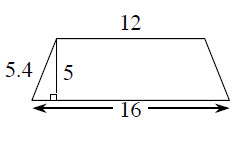2.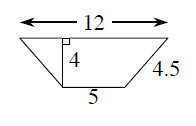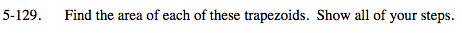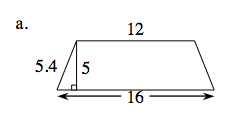The equation for the area of a trapezoid is:

$\text{Area}=\frac{1}{2}(\text{base } 1 + \text{base }2)(\text{height})$

Locate the different parts of the equation on the trapezoid.
Then substitute the values and solve the equation to find the area.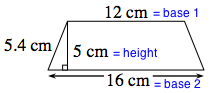$\text{Area}=\frac{1}{2}(12+16)(5)$

Area = 70 square cm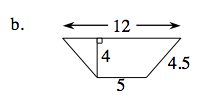See part (a).